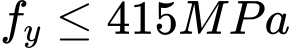The link was sent to your email.

Tree
Settings
Product:
Program:
Language:

## Verification of rectangular RC cross-section

The cross-section is rectangular, unilaterally reinforced and loaded by the bending moment and normal compression force. The program verifies a reinforced concrete section using the method of limit deformation. The maximum allowable strain of concrete in compression is 0.002 - 0.0035. Compression reinforcement is not taken into account. Minimum eccentricity is applied:The computed degree of reinforcement is checked using the following expressions: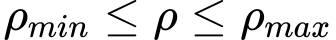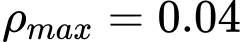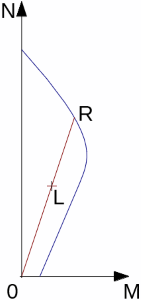Interaction diagram N-M

Usage ratio of concrete cross-section subject to the combination of bending moment and normal force is determined as |0L| / |0R|. Where L is load and R is strength with prescribed excentricity.

## Bending without normal force

The cross-section is rectangular, reinforced on one side and loaded by the bending moment M.

The permissible moment for a given area of reinforcements As reads: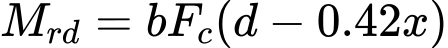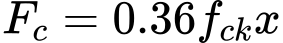The program further checks whether the location of neutral axis x is less than the limit location of neutral axis xmax given by:

 xmax = 0.53d - for steel Fe 250 xmax = 0.48d - for steel Fe 400 xmax = 0.46d - for steel Fe 500

## Shear

First, the program computes the ultimate shear strength of concrete Vuc.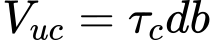where: τc is determined according to table 19 standard IS 456 : 2000.

If the ultimate shear strength of concrete is exceeded, the ultimate shear strength Vuc,max is checked.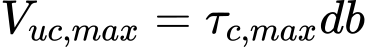where: τc,max is determined according to table 20 standard IS 456 : 2000.

Next, the necessary reinforcement area is given by:where: